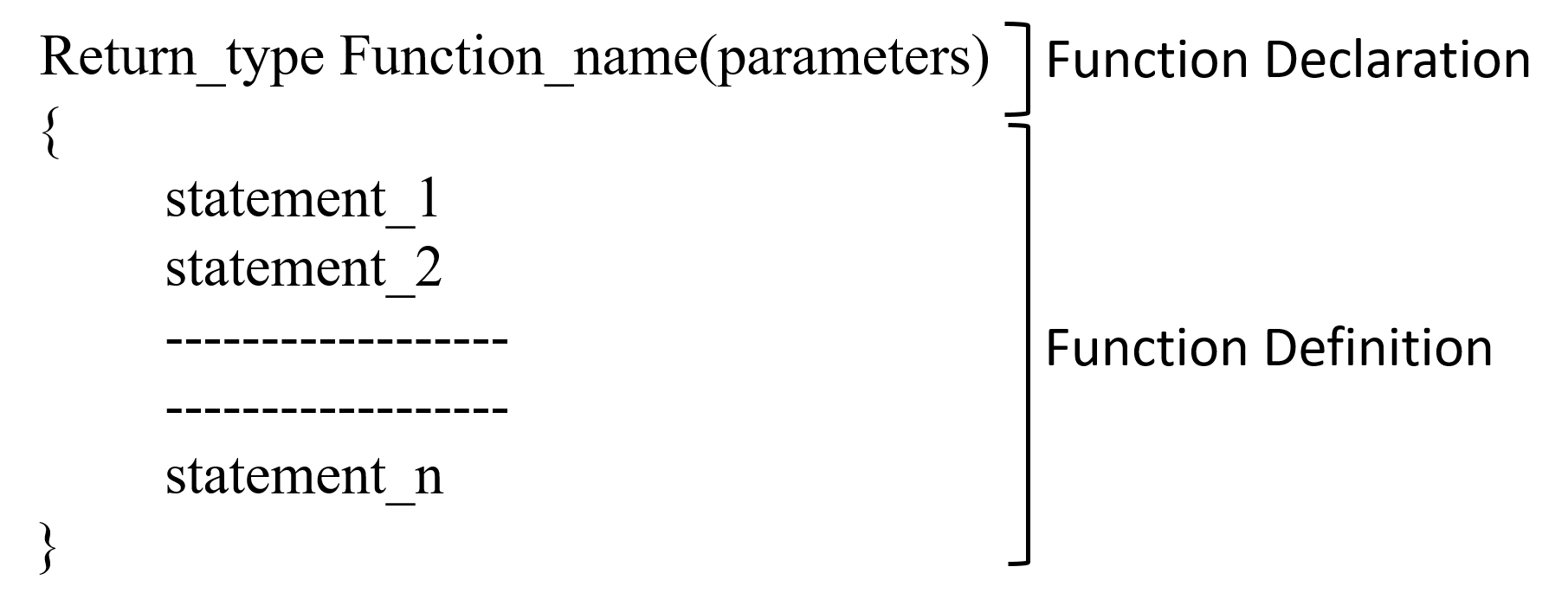# Fifth Chapter Lesson-20: Function in C programming language

## At the end of this lesson-

• 1. You will be able to explain function.
• 2. You will be able to explain library function and user defined function.
• 3. You will be able to explain the importance of using function.
• 4. You will be able to explain recursive function and it’s advantages and disadvantages.

Function: A function is a group of statements that together perform a specific task. A function that take inputs, do some process and produces a output.

### Syntax of a function:Function declaration: A function declaration tells the compiler about a function’s name, return type, and parameters.

Return_type: A function may return a value. Return type can be of any data type such as int, double, char, void, short etc. Some functions perform the desired operations without returning a value. In this case, the return_type is the keyword void.

Function_name: It is an identifier and it can be anything, however it is advised to have a meaningful name for the functions so that it would be easy to understand the purpose of function just by seeing it’s name.To write function’s name rules of identifier should be followed.

Parameters: Parameters contains variables names along with their data types that defines what type and how many inputs for the function. Parameters are optional; that is, a function may contain no parameters.

Function definition:The function body contains a collection of statements that define what the function does.

There are two types of function in C programming:

• 1. Standard library functions
• 2. User defined functions

Standard library functions: The standard library functions are built-in functions in the compiler which already have a definition in header files, so we just call them from main() function whenever there is a need to use them. For example- scanf(), printf(), gets(), puts(), getchar(), putchar(), abs(), pow(b,p), sqrt(), sin(), cos(), tan(), rand() etc. To use each of the library function in the program, programmer should add the respective header file at the top of the program. Library functions are two types. They are-

Numerical function: Perform mathematical operations. eg. pow(b,p), sqrt(), sin(), rand() etc.

String Function: Perform string related operation. eg. strcpy(), strcat(), strcmp() etc.

Some library functions and their respective header file:

 Library function Header file scanf(),printf(), gets(), puts(), getchar(), putchar() sqrt(), pow(), abs(), sin(), cos(), tan(), rand() clrscr(), getch() strcpy(), strcat(), strcmp()

User defined functions: The functions that a programmer create in a program are known as user defined functions. main() function is called user-defined function. Each C program must have at least one function, which is main(). You can create as many user-defined functions as you want.

Functions are used because of following reasons –

1. To improve the readability of code.
2.  Improves the reusability of the code, same function can be used in any program rather than writing the same code from scratch.
3.  Debugging of the code would be easier if you use functions, as errors are easy to be traced.
4.  Reduces the size of the code, duplicate set of statements are replaced by function calls.

Elements of function: The following four things are considered when a user-defined function is used in a ‘C’ program.

• 1. Function definition
• 2. Function Call
• 3. Prototype of function
• 4. Return type and return statement of function

Recursive function: A function can call any other function as well as can call itself. When a function calls itself then the function is called recursive function and the process of calling itself is called recursion. While using recursion, programmers need to be careful to define an exit condition from the function, otherwise it will go into an infinite loop. Recursive functions are very useful to solve many mathematical problems, such as calculating the factorial of a number, generating Fibonacci series, etc.

• 1. Reduce unnecessary calling of function.
• 2. Through recursion one can solve problems in easy way while its iterative solution is very big and complex.
• 3. Extremely useful when applying the same solution.

• 1. Recursive solution is always logical and it is very difficult to debug and understand.
• 2. Fairly slower than its iterative solution.
• 3. For each step we make a recursive call to a function. For which it occupies significant amount of stack memory with each step.

## Lesson Evaluation-

Knowledge Based Questions:

• a. What is function?
• a. What is library function?
• a. What is user-defined function?
• a. What is recursive function?
• a. What is recursion?

Comprehension Based Questions:

• b. ‘Library function and user-defined function in program are not same’- explain.
• b. What type of function is main()? explain.
• b. Header file has to be written for what type of function using?
• b. Why header file has to be written for using library function in program?

Creative Questions:

Multiple Choice Questions:

Written by,